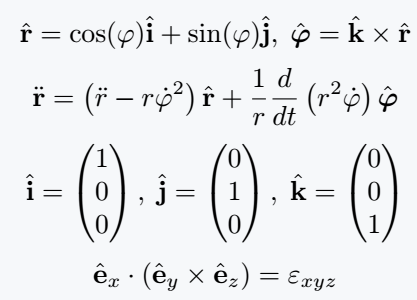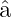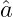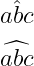# How to write a hat(^) symbol in LaTeX?

Hat is a mathematical notation used in various branches of mathematics and science. Also, the hat symbol is used in languages.

In that case, the hat symbol is usually called a circumflex symbol.

In latex, the hat symbol is used separately in both text and math modes.

Properties Valu
Symbol Hat
Argument Yes(a,u,i,j,k,..etc.)
Command \hat{}
Example \hat{a} → â

## Hat symbol in math mode

LaTeX provides a default command to use the hat symbol in LaTeX’s math mode, which is \hat{}.

If you pass a character as an argument to this command, the hat symbol will be printed over the character in the document.

\documentclass{article}
\usepackage{bm,amsmath}
% bm package for \boldsymbol command
% amsmath package for matrix
\begin{document}
$$\hat{\mathbf{r}} = \cos(\varphi)\hat{\mathbf{i}} + \sin(\varphi)\hat{\mathbf{j}},\; \hat{\boldsymbol{\varphi}} = \hat{\mathbf{k}}\times\hat{\mathbf{r}}$$
$$\ddot{\mathbf{r}} = \left( \ddot{r}-r\dot{\varphi}^2 \right)\hat{\mathbf{r}}+\frac{1}{r}\frac{d}{dt}\left(r^2\dot{\varphi}\right)\hat{\boldsymbol{\varphi}}$$
$$\hat{\mathbf{i}} = \begin{pmatrix} 1 \\ 0 \\ 0 \end{pmatrix},\;\hat{\mathbf{j}} = \begin{pmatrix} 0 \\ 1 \\ 0 \end{pmatrix}, \; \hat{\mathbf{k}} = \begin{pmatrix} 0 \\ 0 \\ 1 \end{pmatrix}$$
$$\hat{\mathbf{e}}_x\cdot(\hat{\mathbf{e}}_y\times\hat{\mathbf{e}}_z)= \varepsilon_{xyz}$$
\end{document}

Output :If you look at the program above, you will understand that for Math mode in Latex, you have to use $$...$$ or $...$ on both sides of the expression.

You can easily solve the above problem using physics package. Because in physics, the hat symbol is used to denote the unit vector.

And you need to use \vu command to define this unit vector.

\documentclass{article}
\usepackage{physics}
\begin{document}
$$\vu{a}$$
$$\vu*{a}$$
\end{document}

Output :If you look at the output above, you will notice that the * symbol is used with the \vu command for the Greek and Italic style.

If you want to use hat symbols on more than one character, you need to use the \widehat{} command instead of the \hat{} command.

\documentclass{article}
\begin{document}
$$\hat{abc}$$
$$\widehat{abc}$$
\end{document}

Output :If you look at the output above, you can see the difference between the two symbols.

## Hat symbol in text mode

This Circumflex symbol is used to write the letters of different mother tongues. For this, you need to use inline \^{ } command.

\documentclass{article}
\begin{document}
Circumflex-\^{o},\^{a}
\end{document}

Output :

Circumflex-ô,â#### Md Jidan Mondal

LaTeX expert with over 10 years of experience in document preparation and typesetting. Specializes in creating professional documents, reports, and presentations using LaTeX.